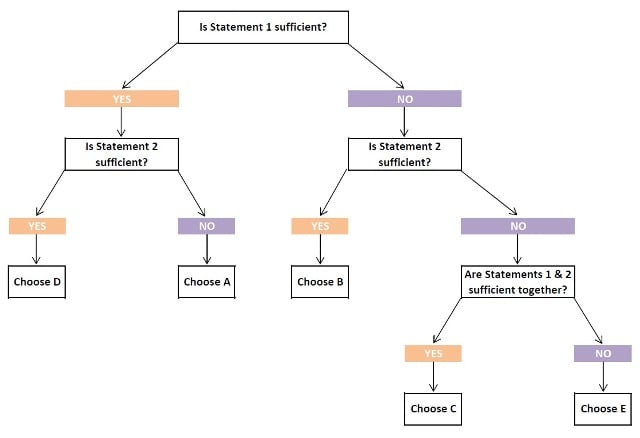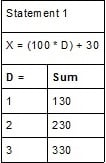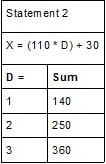GMAT Tips for Quant: Data Sufficiency | TopMBA.com

# GMAT Tips for Quant: Data Sufficiency

Updated April 16, 2021

Over the years I’ve acquired a solid foundation of cooking techniques. From sautéing to broiling, I’m quite comfortable in the kitchen. One dish I can’t cook, however, is crème brûlée. I haven’t tested this hypothesis, but I have a feeling that my blowtorch skills are well below par. To me, crème brûlée is an oddity in the cooking world. It requires skills that aren’t commonly practiced and seems daunting at first glance.

Data sufficiency (DS) questions are the ‘crème brûlée’ of the GMAT. No other entrance examination includes data sufficiency questions or any derivative of them. When studying for quant, you’ll certainly need to review math concepts, but you shouldn’t have to learn how to answer problem solving (PS) questions. Unlike data sufficiency, you’ve been answering those sorts of questions in every math class you’ve taken. To excel at data sufficiency, however, you must rely on strategy.

## Data sufficiency is different

Simply put, the DS section of the GMAT is different. It’s different because you aren’t trying to solve for one answer, like PS. Instead, your goal is to test for sufficiency. That means you’re trying to determine whether or not you’ve been provided enough information to definitively answer the question stem. Take this question stem, for example:

Is X > 9?

(1) X is Positive

(2) X - 2 = 11

To reiterate, you goal isn’t to solve for X, but to determine if the statements provided answer the question stem’s query. In this case, statement 1 is too vague: X could be any number greater than 0. Statement 2, however, tells us that when X is reduced by 2, it produces a number greater than 9. Therefore, statement 2 is sufficient.

Another quirk of DS is that every problem contains the same five answer choices. This should be intuitive: if your goal is proving sufficiency, how many different answers can there be? Something can’t be varying degrees of sufficient, right? Each problem offers two statements used to prove the question stem’s sufficiency. The answer choices mirror this objective:

a) Statement 1 ALONE is sufficient, but statement 2 alone is not sufficient to answer the question asked;

b) Statement 2 ALONE is sufficient, but statement 1 alone is not sufficient to answer the question asked;

c) BOTH statements 1 and 2 TOGETHER are sufficient to answer the question asked, but NEITHER statement ALONE is sufficient.

e) Statements 1 and 2 TOGETHER are NOT sufficient to answer the question asked, and additional data specific to the problem are needed.

Because of its unique nature, DS can be a question type that test takers loathe. At its core, however, there are really only two reasons why you’d get a DS question wrong:

• You think you don’t have all of the information you need to prove sufficiency, but you do. Solution: Maximize the information you do have. Use the question stem, the other statement, simplifying with algebra, etc.
• You think you have all of the information you need to prove sufficiency, but you don’t. Solution: Be skeptical of the information you received and play devil’s advocate; if picking numbers, be sure to choose ones on both sides of the statement.

Despite what you might think at first, DS can actually be a refreshing change-of-pace from PS. Because you’re testing for sufficiency, not solving for one answer, DS problems can take less time to complete. Also, because the answer choices are always the same, DS is ripe with strategic opportunities – which we’ll discuss next.

## GMAT tips: Basic strategy

If you ever hear an interview with a professional athlete about their superstitions, you’ll probably think they’re insane. Athletes obsess over the smallest of details: which shoe they tie first, what to eat before a game, and even where to sit on the team bus and/or plane. While this doesn’t convince the ‘Sports Gods’ to treat them favorably, it does give the athlete a sense of confidence and calm.

Following superstitions makes the athlete feel like they’re doing everything in their power to perform their best. This shouldn’t sound insane, however. Most people follow superstitions, also known as a routine, every day. Whether it’s eating a certain type of breakfast or remembering to pack an umbrella, routines are part of daily life. Again, while this doesn’t mean you’ll have a better life by doing so; routines do provide us with a sense of confidence and calm.

Eating lunch at a certain time each day might not provide you with any tangible benefits, but when approaching the GMAT DS problems, having a routine is extremely important and is the first of my GMAT tips for DS. Because DS is so strategically focused, more so than PS or the three verbal question types, and each question follows a similar pattern, approaching each problem the same way isn’t only possible, but it’s necessary to achieve a high score in quant.

When a DS problem comes up on the exam, before you even read the question, write down ‘AD / BCE’. This will help you keep track of your answers through the decision tree - which we’ll discuss later. Some guides recommend creating a grid before you begin the quant section.

If that’s your preference, feel free to do so. Otherwise, it can be easier to write ‘AD / BCE’ each time to not only engrain your routine, but so that you don’t have to keep flipping back through your scratch paper to find the grid.

After you’ve written down ‘AD / BCE’, read the question stem and ask yourself if it wants you to find the sufficiency of a specific ‘value’ or a ‘yes/no’ response. Depending on what’s asked, the definition of whether a statement is or isn’t sufficient could change.

• A value question will ask you for the exact value of something. If a statement narrows the possibilities down to exactly one number, not a range, it’s sufficient. Therefore, you’ll need to test at least two different numbers.
• A yes/no question will ask whether the question stem is or isn’t correct. For example, “Is X > 10?” Therefore, you should aim to find a yes and a ‘no’ outcome when testing for sufficiency.

Once you’ve determined what type of question you’re tasked with, your next step, and the next of my GMAT tips, is to simplify the question stem and statements. Doing so will make testing for sufficiency, either by algebra or by picking numbers, a much easier process. For example:

Is X >0?

(1) X2 = 9x

(2) X < Y

Focusing on statement 1, x2 = 9x can be simplified into X(X - 9) = 0. Therefore, X = 0, 9. Since there are two possible answers for X, statement 1 doesn’t sufficiently answer the question stem.

Assuming you understand the aim of the question stem and the provided information is simplified, your next step is to test for sufficiency, following the decision tree. The decision tree is, as follows, and begins with statement 1:For the non-visual learner, your first test of sufficiency is statement 1. Depending on whether or not it’s sufficient, you test statement 2 individually and then the two statements together. Because these tests relate to each other, once you find out an individual statement’s sufficiency, you can immediately eliminate other answer choices.

Remember that AD / BCE grid? If statement 1 is sufficient, you can immediately cross off answer choices B, C, and E. You only have to test statement 2 to see if it’s also sufficient. If it is, the answer is D: both statements are individually sufficient. If not, then A is your answer: statement 1 alone is sufficient.

That’s your DS routine. In the next sections we’ll discuss how to test statements for sufficiency and some advanced strategy. To summarize:

1. Write down ‘AD / BCE’

3. Simplify the question stem and statements 1 and 2

• Test statement 1
• Test statement 2
• Test both statements together (if applicable)

5. Choose answer and move to the next question

## Testing for sufficiency

You have three main options to test statements for sufficiency:

• Algebra
• Picking numbers
• Informed guess

Algebra

Breaking down each statement algebraically is the best way to test a statement for sufficiency. Solving algebraically does require a higher understanding of math, but limits careless mistakes one can make using a strategy like picking numbers. The test makers build traps into DS problems and unless you dissect the question stem and statements with algebra, you might fall into one.

Picking numbers

Time is a factor, however, and so if you’re unable to use algebra, picking numbers is an excellent substitute. As explained in the PS post, picking numbers is a type of strategy where the test taker assigns values to the variable(s) listed in the question stem and statements. By doing so, you’re essentially guessing at what might be a solution. You shouldn’t just pick any number, however. Pick ‘smart’ numbers, meaning ones that could prove both sides of the statement. A commonly used set is every half-number from negative two to positive two:

• {-2, -3/2, -1, -1/2, 0, 1/2, 1, 3/2, 2}

When picking numbers, make sure you cast a wide net. To do so, you’ll need to select at least two sets of options. For example, a question stem with two variables might be tested with {-1, 0} and then {1/2, 2}. To those without a strong command of algebra, fear not. A mix of algebra and picking numbers can still be a successful plan of attack.

Informed guess

Even if you apply the right strategy, avoid traps set by the test makers, and follow the above GMAT tips, you still might not feel confident enough to answer the question. In that situation, you’ll have to make an informed guess. The key to guessing in DS, and the GMAT as a whole, is to try to increase your odds of answering correctly: it’s far better to guess between two answers than five.

To improve your odds, try to evaluate at least one of the statements in the decision tree. This way you’ll eliminate at least two answer choices through ‘AD / BCE’. Try to resist the urge to guess E. In general, complicated or hard-to-evaluate statements are more likely to be sufficient than insufficient. If you think E is correct, however, to be safe, make sure to prove it out algebraically.

As discussed in the above GMAT tips, a quirk of DS is that you don’t necessarily need to solve the entire problem to know the correct answer. You only need to determine if the statement answers the question stem or not. In some case, this means you can complete DS problems at a faster rate than PS. With speed comes careless mistakes, however, so here are a few of the most common tricks the test makers use:

• Assumption - Assuming something about a statement or question stem. If it isn’t disclosed, don’t assume. For example, don't assume that a value must be an integer or must be a positive number.
• Contradiction - Statements 1 & 2 must come to the same conclusion. If they don’t, you messed up the math. That means if statement 1 says X = 2 and statement 2 says X = 4, you need to start over. That simply isn't possible.
• The N rule - If you have N unique variables, you need N distinct equations to solve for them. Knowing this property can help you answer more difficult DS problems.
• Identical equations - Equations that simplify into themselves. You may think that X = 2 and 2X = 4 are different equations, but they simplify into themselves. Be careful to not misinterpret them and select the wrong answer. If this is the case, you know that the correct answer is only D or E. Either each statement is sufficient, or they’re both insufficient.
• Beware of 0 - Don’t forget that 0 is an integer, and when you’re picking numbers, it can be a smart choice. Further, whenever dividing a small number by a bigger one, remember that the quotient is always 0 and the remainder is the divisor.
• No is yes - While rare, if a statement consistently answers the question stem as ‘no’, then that statement is sufficient. Don’t get confused by the fact that the yes/no objective is fulfilled by no. No can answer the question stem.
• The C trap - The test makers know you’d prefer to have more information, so if C is clearly sufficient and A & B don’t seem to be individually sufficient, reexamine. Chances are it’s a trap. Try to prove out C before selecting it.
• Be skeptical - Always be skeptical of the test makers. If an answer seems too easy, it probably is. If it was that easy, the question would be in the 20th percentile. Since you are testing way above that range, don’t fall for the trap.

To build off the ‘C trap’ and ‘be skeptical’ points, if one statement is obviously sufficient, then the other must be included for a reason. Either it will give you context for the first statement, or it will show you something you overlooked. While you need to test each statement individually, you don’t need to do it in a vacuum. Use all of the clues available. For example:

How many trees are pine in a grove of 30 pine, oak, and maple trees?

(1) The ratio of oak to maple trees is 14:9

(2) The ratio of oak to pine trees is 2:1

At first glance you might think this is an easy question in which you can find the answer through both statements - choice C. And while that’s true, both statements aren’t necessary to answer this question correctly. In fact, the right answer is A. If there are 30 trees total and the ratio of oak to maple is 14:9, there can only be room for seven more trees: Statement 1 is sufficient.

## Putting it all together

Let’s illustrate our routine and the strategies discussed above with a practice question:

What is the tens digit of positive integer X?

(1) X divided by 100 has a remainder of 30

(2) X divided by 110 has a remainder of 30

At first glance, what does this question tell us? A quick observation is that:

• This is a ‘value’ question
• X is a positive integer
• It deals with number properties
• It’s a ‘remainder’ problem

Knowing that X is a positive integer is particularly helpful when picking numbers. Also, knowing the equation for remainders will allow us to solve this question quickly. Specifically, that equation states: any number X is the sum of the dividend multiplied by the divisor plus the remainder.

Translating math to English, the equation for statements 1 and 2 would be:

• X = 100*D + 30
• X = 110*D + 30

The goal of this question is to find the tens digit of X. Let's pick some numbers and see if we can see a pattern in statement 1:Clearly, statement 1 is sufficient. The tens digit, regardless of the divisor, is always 3. From here we can eliminate B, C, and E. The only other possible answer choice is D – that is, if statement 2 is also sufficient. Let's find out:As you can see, the tens digit changes with each different divisor. This is a ‘value’ question, so only one answer can possibly make the question prompt sufficient. Since that isn't the case in statement 2, it's insufficient; the correct answer is A.

***

That’s really all of the strategy you’ll need for DS. If you keep that in mind and remember to follow your routine, you’ll be ready to score your best. While DS seems daunting at first, with practice, it’ll be as easy as crème brûlée…

This article was originally published in May 2015 . It was last updated in April 2021